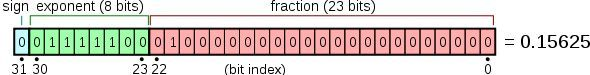### IEEE 754

IEEE二进制浮点数算术标准（IEEE 754）是20世纪80年代以来最广泛使用的浮点数运算标准，为许多CPU与浮点运算器所采用。这个标准定义了表示浮点数的格式（包括负零-0）与反常值（denormal number）），一些特殊数值（无穷（Inf）与非数值（NaN）），以及这些数值的“浮点数运算符”；它也指明了四种数值舍入规则和五种例外状况（包括例外发生的时机与处理方式）。• 单精度 8 位： $2^{8-1}-1$ = 127
• 双精度 11 位： $2^{11-1}-1$ = 1023

• 单精度 23 位
• 双精度 52 位

### 转二进制

1. 先转整数部分（除 2 取余）

• 4 / 2 = 2 余 0
• 2 / 2 = 1 余 0
• 1 / 2 = 1 余 1

4 = 100

2. 再转小数部分（乘 2 取整）

• 0.375 * 2 = 0.75 记 0
• 0.75 * 2 = 1.5 记 1
• 0.5 * 2 = 1 记 1

0.375 = 011

0.2 转 2 进制：0.00110011001100110011001100110011001100110011…..

### 转回十进制

V = (-1)^s *（1+M）* 2^(E-127)（单精度）
V = (-1)^s *（1+M）* 2^(E-1023)（双精度）
s: 标志位
M: 有效数值
E: 指数偏移值

$(-1)^0$ * (1 + 5033165 * $2^{-23}$) * $2^{(124-127)}$ ≈ 0.20000000298023